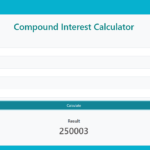## Compound Interest Calculator In JavaScript With Source Code

Compound Interest Calculator is a simple project in HTML5, CSS, and JavaScript. This is an amazing calculator made for the user convenient, to help them find out about the addition of interest to the principal sum of a loan or deposit. This project is for...

Type : Project

File Size : 5.7 KB# Similar Projects and Reports

#### Compound Interest Calculator In JavaScript With Source Code

Compound Interest Calculator is a simple project in HTML5, CSS, and JavaScript. This is an amazing calculator made for the user convenient, to help them find out about the addition of interest to the principal sum of a loan or deposit. This project is for...

#### Simple Interest Calculator In JavaScript With Source Code

Simple Interest Calculator is a simple project in HTML5, CSS, and JavaScript. This is an amazing calculator made for the user convenient, to help them calculate out the total amount of interest to the principal sum of a loan or deposit. This project is fo...

#### Compound Interest Calculator In C# With Source Code

Compound Interest Calculator in C# is based on the concept for the user convenience, to help them find out about the addition of interest to the principal sum of a loan or deposit. This project is for financial calculating to figure out the loan’s payment...

#### Calculator In JavaScript With Source Code

The calculator is a simple project in HTML5, CSS, and JavaScript. Here, the calculator functions as that of a real-life simple calculator. This project is for solving the mathematical calculations of numbers. You can see the basic features of the calculat...

#### Web Calculator In JavaScript with Source Code

Web Calculator is a simple project in HTML5, CSS, and JavaScript. This project is for solving the mathematical calculations of numbers. You can see the basic features of the advanced calculator in this project. Also, there are two modes in this calculator...

#### GPA Calculator In JavaScript With Source Code

GPA Calculator is a simple project in HTML5, CSS, and JavaScript. This is an interesting project. This project is for calculating the total marks, percentage, grade, and GPA of the student. this very useful project for schools and colleges. The user can e...